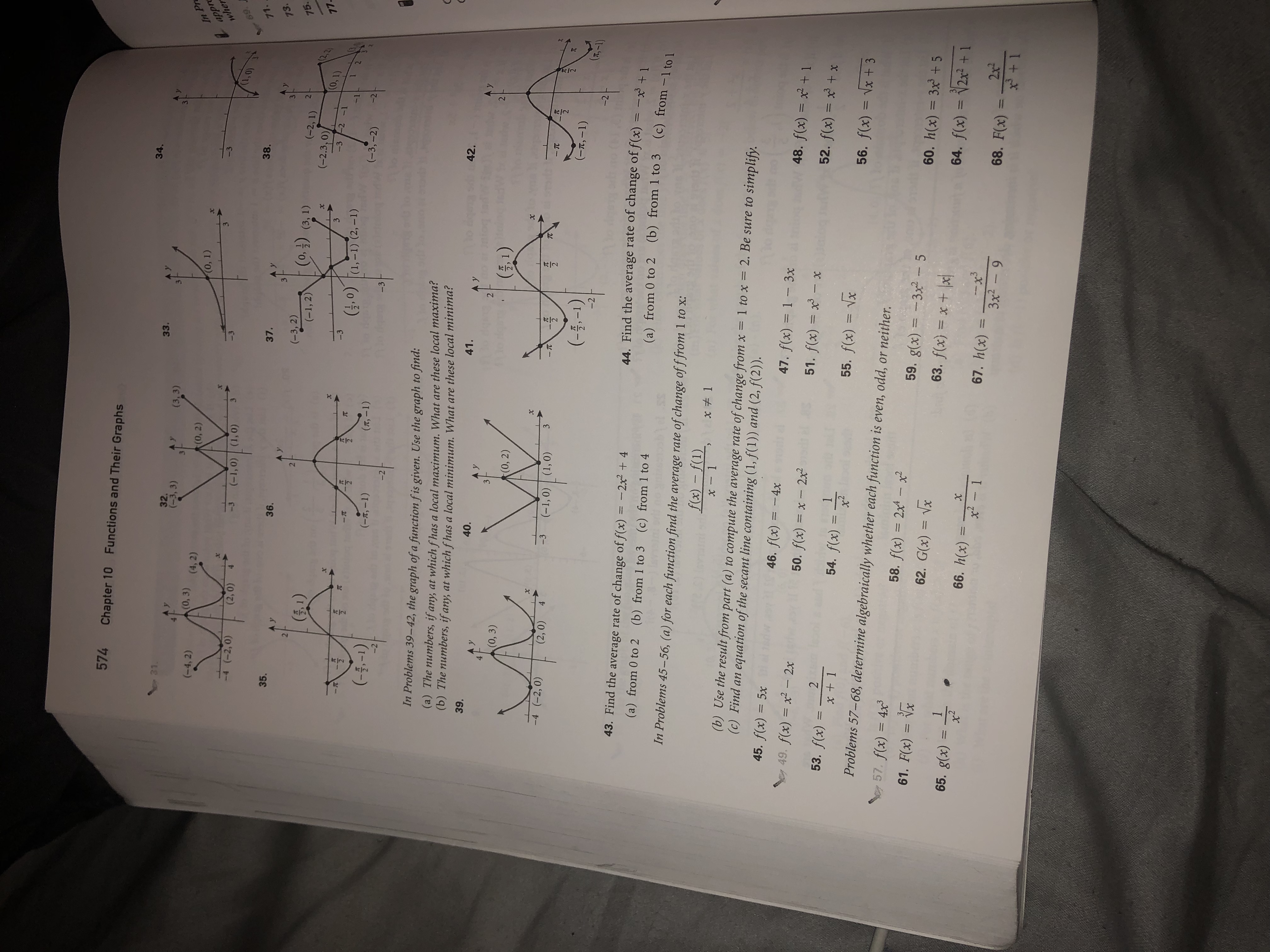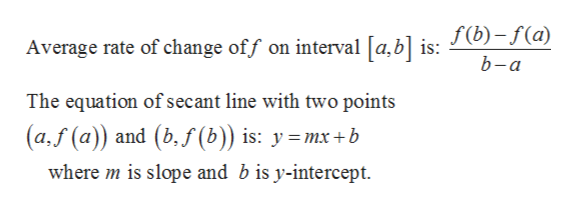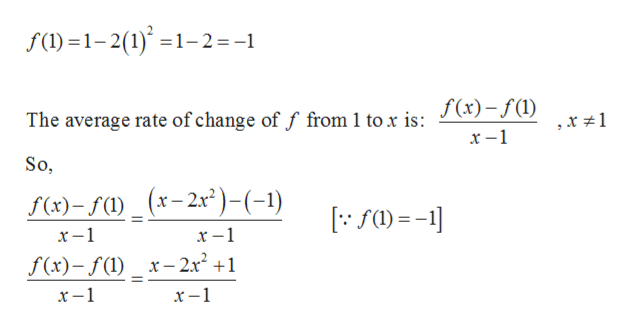574Chapter 10Functions and Their Graphs34.- 31.33.32.(-3, 3)(3,3)In Prapprwher(0, 3) (4,2)(-4, 2)(0, 2)(0, 1)-4 (-2,0)(2,0)(-1, 0) (1,0)-3(1,0)6971.38.37.35.A Y36.A y73.(-3, 2)(-1, 2)(0,3) (3, 1)(1)2(-2, 1)75.(2.2)(0,1)(-2.3,0)X77X-3-2 -1-3,0) (1,-1) (2, -1)-1(--1)(-3,-2)(T,-1)(-t,-1)-3-2-2In Problems 39-42, the graph of a function fis given. Use the graph to find:(a) The numbers, if any, at which fhas a local maximum. What are these local maxima(b) The numbers, if any, at which fhas a local minimum. What are these local minima42.39.41.40.A yA Y2143(0, 3)(0, 2)Xx-4 (-2, 0)(2,0)TC-3(-1,0) (1,0)43-TC-TC2(-5-1)(r,-1)T,-1)-2-243. Find the average rate of change of f(x) = -2x + 444. Find the average rate of change of f(x) = -(a) from 0 to 2 (b) from 1 to 3 (c) from 1 to 4(b) from 1 to 3(a) from 0 to 2(c) from -1 to 1In Problems 45-56, (a) for each function find the average rate of change of f from 1 to x:f(x)-f(1)x 1x-1(b) Use the result from part (a) to compute the average rate of change from x = 1 to x = 2. Be sure to simplify.(c) Find an equation of the secant line containing (1, f(1)) and (2, f(2)).45. f(x) = 5x46. f(x) 4x47. f(x) 1 3x48. f(x) = +149. f(x) x2- 2x50. f(x) x - 2x251. f(x) x- x52. f(x) = x +x253. f(x)154. f(x) =x + 155. f(x) xx2Problems 57-68, determine algebraically whether each function is even, odd, or neither.56. f(x) = \x + 357. f(x) = 4x358. f(x) 2x4 - x59. g(x)61. F(x) = Vx=- 3x2- 560. h(x) = 3x +562. G(x)= Vx63. f(x) x+165. g(x)x264. f(x) = 2x +166. h(x)x2xx33x2-967. h(x)12x268. F(x)11x3 + 1

Question

Do not know how to do question 50 on this page in the book, plz show steps toohelp_outlineImage Transcriptionclose574 Chapter 10 Functions and Their Graphs 34. - 31. 33. 32. (-3, 3) (3,3) In Pr appr wher (0, 3) (4,2) (-4, 2) (0, 2) (0, 1) -4 (-2,0) (2,0) (-1, 0) (1,0) -3 (1,0) 69 71. 38. 37. 35. A Y 36. A y 73. (-3, 2) (-1, 2) (0,3) (3, 1) (1) 2 (-2, 1) 75. (2.2) (0,1) (-2.3,0) X 77 X -3-2 -1 -3 ,0) (1,-1) (2, -1) -1 (--1) (-3,-2) (T,-1) (-t,-1) -3 -2 -2 In Problems 39-42, the graph of a function fis given. Use the graph to find: (a) The numbers, if any, at which fhas a local maximum. What are these local maxima (b) The numbers, if any, at which fhas a local minimum. What are these local minima 42. 39. 41. 40. A y A Y 21 4 3 (0, 3) (0, 2) Xx -4 (-2, 0) (2,0) TC -3 (-1,0) (1,0) 4 3 -TC -TC 2 (-5-1) (r,-1) T,-1) -2 -2 43. Find the average rate of change of f(x) = -2x + 4 44. Find the average rate of change of f(x) = - (a) from 0 to 2 (b) from 1 to 3 (c) from 1 to 4 (b) from 1 to 3 (a) from 0 to 2 (c) from -1 to 1 In Problems 45-56, (a) for each function find the average rate of change of f from 1 to x: f(x)-f(1) x 1 x-1 (b) Use the result from part (a) to compute the average rate of change from x = 1 to x = 2. Be sure to simplify. (c) Find an equation of the secant line containing (1, f(1)) and (2, f(2)). 45. f(x) = 5x 46. f(x) 4x 47. f(x) 1 3x 48. f(x) = +1 49. f(x) x2- 2x 50. f(x) x - 2x2 51. f(x) x- x 52. f(x) = x +x 2 53. f(x) 1 54. f(x) = x + 1 55. f(x) x x2 Problems 57-68, determine algebraically whether each function is even, odd, or neither. 56. f(x) = \x + 3 57. f(x) = 4x3 58. f(x) 2x4 - x 59. g(x) 61. F(x) = Vx = - 3x2- 5 60. h(x) = 3x +5 62. G(x) = Vx 63. f(x) x+ 1 65. g(x) x2 64. f(x) = 2x +1 66. h(x) x2 x x3 3x2-9 67. h(x) 1 2x2 68. F(x) 11 x3 + 1 fullscreen
Step 1

Given:

Step 2

Concept used:help_outlineImage TranscriptioncloseAverage rate of change off on interval [a,b] is: J(b)-f(a) b-a The equation of secant line with two points (a,f (a)) and (b,f(b)) is: ymx+b where m is slope and b is y-intercept fullscreen
Step 3

Part(a):...help_outlineImage Transcriptionclosef(l)1-2(1)1-2=-1 The average rate of change of f from 1 to x is: J(x)-f(1) So, f(x)-f()_(x-2x)-(-1) х —1 х —1 f(x)-f() x-2x2 +1 х —1 х —1 fullscreen

Want to see the full answer?

See Solution

Want to see this answer and more?

Our solutions are written by experts, many with advanced degrees, and available 24/7

See Solution
Tagged in

Algebra